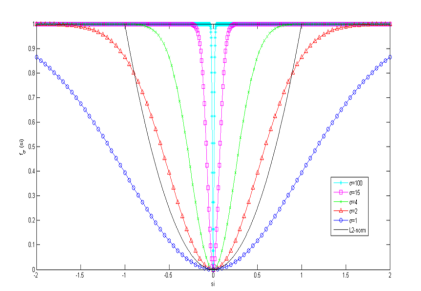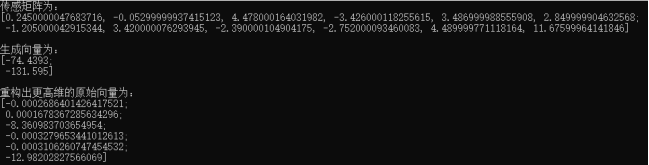• 本工具箱包含常用的压缩感知图像重构算法，如OMP,BP,IHT,等算法，非常齐全。
• 针对已有压缩感知重构算法重构精度不高、消耗时间长的问题，在研究[lp]范数和光滑[l0]范数压缩感知重构算法的基础上提出改进算法。通过极大熵函数构造一种光滑函数来逼近最小[lp] 范数，对解序列进行离散化来近似...
• Matelab 编程CS图像重构算法怎么输出均方差、峰值信噪比等，有没有谁给我完整的程序，让我做完毕业设计
• 压缩感知OMP重构算法matlab实现，OMP重构算法，本程序用于重构原始图像
• 压缩感知中经典的StOMP重构算法，代码含有详细的中文注释，易懂，并且也出了重构图像评价质量标准
• 针对此缺陷,提出一种用于高光谱图像的埃尔米特压缩感知重构算法,主要思想是:利用埃尔米特求逆引理,对正交匹配追踪算法残差更新的迭代过程进行优化;进一步地,采用人工鱼群算法寻找最优原子,对匹配过程进行加速,以提高...
• 针对传统压缩感知在核磁共振成像中存在着重构算法慢、成像时间长的缺点, 利用核磁共振图像自身非满秩的特点, 在压缩感知框架下以奇异值分解作为基底对图像稀疏表示进行了研究, 并对重构算法进行了优化。实验结果表明...
• 二维图像（二维图像压缩感知重构算法程序代码、内含完整的MATLAB代码）
• 针对当前重建效果最好的基于低秩先验的NLR重建算法，忽略了图像的局部结构信息，不能有效地重建图像的边缘，为了在...实验结果表明，与传统的稀疏性先验重建算法和NLR算法相比，所提算法能够获得更高的图像重构质量。稀疏表示
• 为了提高现有块压缩感知重构算法的性能，提出了基于全变分和混合变分模型的块压缩感知（简称BCS-TV和BCS-MV）算法。该方法以块为单位进行图像采样，以自然图像正则项的稀疏性为先验条件，通过变型的增广拉格朗日交替...交替方向乘子法
• 随着压缩感知技术的发展, 基于压缩感知图像融合技术... 然后利用一种简单的绝对值最大融合规则直接在压缩感知域进行融合, 最后通过最小全变分的方法重构融合图像。主客观实验结果表明, 该算法具有良好的融合效果。
• 信号分解的稀疏程度决定了压缩感知重构信号的精度，针对标准正交基稀疏程度的不足，提出了基于混合字典的压缩感知图像分解和重构方法。构建匹配图像边缘和纹理的二维Gabor字典，将图像在离散余弦字典与建立的二维...
• 为了能够有效地改善低码率压缩图像的主客观质量，减少图像复原所需观测数据量，节约存储空间和计算量，提出了一种基于多层小波变换的压缩感知图像快速复原算法。该算法将压缩感知理论中的信号重构方法运用于图像复原...
• 为了克服传统的压缩感知重构中正交小波方向选择性差的局限性，针对图像信号方向性决定了需要 在不同纹理区域选择滤波器以使变换后信号能量更加稀疏，提出一种基于自适应方向提升稀疏表示的重构方法。 重构时，...
• # DCT基作为稀疏基，重建算法为OMP算法图像按列进行处理 # 导入所需的第三方库文件 import numpy as np import math from PIL import Image #读取图像，并变成numpy类型的 array im = np.array(Image.open('/...
#coding:utf-8
# DCT基作为稀疏基，重建算法为OMP算法 ，图像按列进行处理
# 导入所需的第三方库文件
import  numpy as np
import math
from PIL import Image

#读取图像，并变成numpy类型的 array
im = np.array(Image.open('/Users/sanfordzhu/Desktop/lena.bmp')) #图片大小256*256

#生成高斯随机测量矩阵
sampleRate=0.5  #采样率
Phi=np.random.randn(int(256*sampleRate),256)

#生成稀疏基DCT矩阵
mat_dct_1d=np.zeros((256,256))
v=range(256)
for k in range(0,256):
dct_1d=np.cos(np.dot(v,k*math.pi/256))
if k>0:
dct_1d=dct_1d-np.mean(dct_1d)
mat_dct_1d[:,k]=dct_1d/np.linalg.norm(dct_1d)

#随机测量
img_cs_1d=np.dot(Phi,im)

#OMP算法函数
def cs_omp(y,D):#传入参数为y和传感矩阵
L=math.floor(y.shape/4)
residual=y  #初始化残差
index=np.zeros((L),dtype=int)
for i in range(L):
index[i]= -1
result=np.zeros((256))
for j in range(L):  #迭代次数
product=np.fabs(np.dot(D.T,residual))
pos=np.argmax(product)  #最大投影系数对应的位置
index[j]=pos
tmp=[]
for tt in range(len(index)):
if (index[tt]>0)or(index[tt]==0):
tmp.append(tt)
tmp1=D[:,tmp]
my = np.linalg.pinv(D[:,tmp])  # 最小二乘
a=np.dot(my,y) #最小二乘
residual=y-np.dot(D[:,tmp],a)
result[tmp]=a
return  result

#重建
sparse_rec_1d=np.zeros((256,256))   # 初始化稀疏系数矩阵
Theta_1d=np.dot(Phi,mat_dct_1d)   #测量矩阵乘上基矩阵
for i in range(256):
print('正在重建第',i,'列。')
column_rec=cs_omp(img_cs_1d[:,i],Theta_1d) #利用OMP算法计算稀疏系数
sparse_rec_1d[:,i]=column_rec;
img_rec=np.dot(mat_dct_1d,sparse_rec_1d)          #稀疏系数乘上基矩阵

#显示重建后的图片
image2=Image.fromarray(img_rec)
image2.show()


展开全文• 针对通过外部学习进行超分辨率存在图像质量不佳、细节不真实的问题提出一种压缩感知和相似性约束的单帧图像超分辨率算法算法首先利用压缩感知中测量域与频域的线性关系对训练库图像在测量域分类，对不同类别图像块...超分辨率
• 针对传统前向后向匹配追踪（FBP）算法运行时间较长的问题，提出了一种自适应加速前向后向匹配追踪（AAFBP）算法。...一维稀疏信号和二维图像的仿真结果表明，AAFBP算法重构精度和运算时间上都更具有优势。自适应阈值
• 压缩感测（CS）理论具有从稀疏视图投影数据重建CT图像的巨大潜力。当前，基于全变异（CT）的CT重建方法是医学CT领域的研究热点，其在迭代过程中使用梯度算子作为稀疏表示方法。但是，用这种方法重建的图像经常会出现...研究论文
• 压缩感知框架下运用正则化正交匹配追踪(ROMP)算法进行图像重构时,迭代次数取值不合适会严重降低重构图像的质量。针对这一问题,提出了确定合理迭代次数的方法。将以往迭代得出的结果作为先验知识,获取具有不同稀疏...
• 图像压缩感知重建中，针对重构效果和耗时不能兼得的问题进行深入研究。基于小波域稀疏，选用常规观测矩阵进行观测采样，通过对观测结果预定义滤波、选取信号硬阈值，引入共轭梯度下降算法，对分段正交匹配追踪...共轭梯度
• 将抗混叠的Contourlet变换应用到基于压缩感知理论的矿井图像重构算法中。仿真实验表明，在相同的观测系统下采用OMP算法对矿井图像进行重建时，相比于传统的Contourlet变换和Sym4小波变换，基于抗混叠Contourlet变换...
• 最近发现网上压缩感知中用OMP算法重构图像的代码很多，但很少有应用OMP算法重构整个视频序列的，代码是自己写的，希望对初入门压缩感知的有帮助。由于重构时间的原因，程序中只对前8帧进行了重构
• 最近发现网上压缩感知中用OMP算法重构图像的代码很多，但很少有应用OMP算法重构整个视频序列的，代码是自己写的，希望对初入门压缩感知的有帮助。由于重构时间的原因，程序中只对前8帧进行了重构
• 关于SAMP算法的实现，大多数采用的是莱斯大学的工具箱中的samp或者CSDN彬彬有礼中的CS_SAMP算法。 rice大学的工具箱中的samp算法实现如下： function [xr iter_num] = SAMP(y, Phi, step_size, sigma); % SAMP: ...
关于SAMP算法的实现，大多数采用的是莱斯大学的工具箱中的samp或者CSDN彬彬有礼中的CS_SAMP算法。

rice大学的工具箱中的samp算法实现如下：

function [xr iter_num] = SAMP(y, Phi, step_size, sigma);
% SAMP: Sparsity Adaptive Matching Pursuit algoritm for compressed sensing.
% For theoretical analysis, please refer to the paper :
% Thong. T. Do, Lu Gan and Trac D. Tran ,"Sparsity Adaptive Matching
% Purusit for practical compressed sensing" available at http://dsp.ece.rice.edu/cs

% Written by Thong Do(thongdo@jhu.edu)
% Updated on July, 26th 2008

% parameter usage:
%   y: Mx1 observation vector      表测量值
%   Phi: MxN measurement matrix    感知矩阵，H
%   step_size: any positive integer value not larger than sparsity    初始步长（0，k）
%   sigma: noise energy when sensing   重构误差
%   xr: reconstructed sparse signal   重构的稀疏表示
%   iter_num: number of iterations    迭代次数

% Initialization
iter_num = 0;             %迭代次数
actset_size = step_size;  %步长
active_set = [];          %支撑集
res = y;                  %残差
stg_idx = 1;              % stage index   阶段1 分阶段进行的

while (norm(res)>sigma)

% candidate list   候选集
[val idx] = sort(abs(Phi'*res), 'descend');       %计算内积并进行降序排序；并记录其对应的列号
candidate_set = [active_set; idx(1:actset_size)];   % 候选集=上一次的支撑集+此次内积的 前 step_size 个对应的列号 ，（即与y相关度最高的step_size个对应的H的列号）

% finalist
[val idx] = sort(abs(pinv(Phi(:,candidate_set))*y), 'descend');   %候选集中的列进行最小二乘（即求X的稀疏估计 X^），并进行降序排序；
new_active_set = candidate_set(idx(1:actset_size));                %选出X^的前actset_size(当前步长)个作为支撑集
new_res = y-Phi(:,new_active_set)*pinv(Phi(:,new_active_set))*y;  % 选出的支撑集 最小二乘进行新的估计并更新残差

if (norm(new_res) >= norm(res))
% shift into a new stage
stg_idx = stg_idx + 1;
actset_size = stg_idx*step_size;

else
% update residual and active set
res = new_res;
active_set= new_active_set;

end

iter_num = iter_num +1;

end % loop

% reconstruction
N = size(Phi,2);
xr = zeros(N,1);
xr_active_set = pinv(Phi(:,active_set))*y;              %选好最终的支撑集进行最小二乘，得到原始信号的估计
xr(active_set) = xr_active_set;


彬彬有礼的SAMP实现如下：

function [ theta ] = CS_SAMP( y,A,S )
%CS_SAMP Summary of this function goes here
%Version: 1.0 written by jbb0523 @2015-05-08
%   Detailed explanation goes here
%   y = Phi * x
%   x = Psi * theta
%	y = Phi*Psi * theta
%   令 A = Phi*Psi, 则y=A*theta
%   现在已知y和A，求theta
%   Reference:Thong T.Do，Lu Gan，Nam Nguyen，Trac D.Tran．Sparsity adaptive
%   matching pursuit algorithm for practical compressed sensing[C]．Asilomar
%   Conference on Signals，Systems，and Computers，Pacific Grove，California，
%   2008，10：581-587.
%   Available at:
%   http://dsp.rice.edu/sites/dsp.rice.edu/files/cs/asilomar08_final.pdf
[y_rows,y_columns] = size(y);
if y_rows<y_columns
y = y';%y should be a column vector
end
[M,N] = size(A);%传感矩阵A为M*N矩阵
theta = zeros(N,1);%用来存储恢复的theta(列向量)
Pos_theta = [];%用来迭代过程中存储A被选择的列序号
r_n = y;%初始化残差(residual)为y
L = S;%初始化步长(Size of the finalist in the first stage)
Stage = 1;%初始化Stage
IterMax = M;
for ii=1:IterMax%最多迭代M次
%(1)Preliminary Test
product = A'*r_n;%传感矩阵A各列与残差的内积
[val,pos]=sort(abs(product),'descend');%降序排列
Sk = pos(1:L);%选出最大的L个
%(2)Make Candidate List
Ck = union(Pos_theta,Sk);
%(3)Final Test
if length(Ck)<=M
At = A(:,Ck);%将A的这几列组成矩阵At
else
theta_ls=0;
break;
end
%y=At*theta，以下求theta的最小二乘解(Least Square)
theta_ls = (At'*At)^(-1)*At'*y;%最小二乘解
[val,pos]=sort(abs(theta_ls),'descend');%降序排列
F = Ck(pos(1:L));
%(4)Compute Residue
%A(:,F)*theta_ls是y在A(:,F)列空间上的正交投影
theta_ls = (A(:,F)'*A(:,F))^(-1)*A(:,F)'*y;
r_new = y - A(:,F)*theta_ls;%更新残差r
if norm(r_new)<1e-6%halting condition true
Pos_theta = F;
%r_n = r_new;%更新r_n以便输出最新的r_n
break;%quit the iteration
elseif norm(r_new)>=norm(r_n)%stage switching
Stage = Stage + 1;%Update the stage index
L = Stage*S;%Update the size of finalist
if ii == IterMax%最后一次循环
Pos_theta = F;%更新Pos_theta以与theta_ls匹配，防止报错
end
%ii = ii - 1;%迭代次数不更新
else
Pos_theta = F;%Update the finalist Fk
r_n = r_new;%Update the residue
end
end
theta(Pos_theta)=theta_ls;%恢复出的theta
end
---------------------
作者：jbb0523
来源：CSDN
原文：https://blog.csdn.net/jbb0523/article/details/45690421
版权声明：本文为博主原创文章，转载请附上博文链接！

但我自己用这两个程序进行图像重构的时候发现重构质量很差，只有在步长为1的时候能较好的恢复图像。寻找了很长时间原因，最终解决了问题。原因如下：

我们从压缩感知最小二乘的求解方式说起，最小二乘用于求解超定方程问题，所以使用的条件应该是所选原子的个数不能超过原子的维度，如我们观测矩阵选择大小为128×256的高斯随机矩阵，那么每个原子的维度是128×1，则我们支撑集中的原子数量不能超过128。在SAMP用于一维信号的重构过程中，由于一维信号是严格稀疏信号，所以一定会达到设定的阈值从而算法收敛。而图像信号是可压缩信号，不是严格稀疏的，所以在算法迭代过程中，终止阈值的选择以及最小二乘使用条件的限制是必必不可少的。

解决方案：

由于算法无法很精确的重构图像信号（无法到达预设的精度，但是算法依旧在执行，导致支撑集中的原子数量一致增加，直到超出了观测矩阵的行数，导致无法使用最小二乘进行求解，导致重构失败），所以终止阈值设置为1e-6是不合理的，可以根据情况设置为500，或者1000，在我的测试实验中，采用DWT作为稀疏基底，高斯随机矩阵作为观测矩阵的时候，阈值设置1000，可以获得较好的效果。

同时我们为了避免最小二乘的使用条件失效，应当在程序中加入以下判断语句。加入之后可以通过调小终止阈值来适当的提升算法精度，因为如果没有以下条件限制，有可能会出现原子数量已经超过观测矩阵的行数但是残差依然没有达到终止阈值的情况，这也是导致重构失败的一个重要原因。

    if length(candidate_set)>=M%候选集合大于了观测值，跳出循环
break;
end

以下是我自己用的SAMP算法重构图像的程序：

function xr = SAMP(y, Phi, step_size, sigma);
% SAMP: Sparsity Adaptive Matching Pursuit algoritm for compressed sensing.
% For theoretical analysis, please refer to the paper :
% Thong. T. Do, Lu Gan and Trac D. Tran ,"Sparsity Adaptive Matching
% Purusit for practical compressed sensing" available at http://dsp.ece.rice.edu/cs

% Written by Thong Do(thongdo@jhu.edu)
% Updated on July, 26th 2008

% parameter usage:
%   y: Mx1 observation vector      表测量值
%   Phi: MxN measurement matrix    感知矩阵，H
%   step_size: any positive integer value not larger than sparsity    初始步长（0，k）
%   sigma: noise energy when sensing   重构误差
%   xr: reconstructed sparse signal   重构的稀疏表示
%   iter_num: number of iterations    迭代次数

% Initialization
M=size(Phi,1);
iter_num = 0;             %迭代次数
actset_size = step_size;  %步长
active_set = [];          %支撑集
res = y;                  %残差
stg_idx = 1;              % stage index   阶段1 分阶段进行的

while (norm(res)>sigma)

% candidate list   候选集
[val idx] = sort(abs(Phi'*res), 'descend');       %计算内积并进行降序排序；并记录其对应的列号
candidate_set = union(active_set,idx(1:actset_size));   % 候选集=上一次的支撑集+此次内积的 前 step_size 个对应的列号 ，（即与y相关度最高的step_size个对应的H的列号）
if length(candidate_set)>=M%候选集合大于了观测值，跳出循环
break;
end
% finalist
[val idx] = sort(abs(pinv(Phi(:,candidate_set))*y), 'descend');   %候选集中的列进行最小二乘（即求X的稀疏估计 X^），并进行降序排序；
new_active_set = candidate_set(idx(1:actset_size));                %选出X^的前actset_size(当前步长)个作为支撑集
new_res = y-Phi(:,new_active_set)*pinv(Phi(:,new_active_set))*y;  % 选出的支撑集 最小二乘进行新的估计并更新残差
norm_new_res=norm(new_res);
norm_res=norm(res);
if (norm(new_res) >= norm(res))
% shift into a new stage
stg_idx = stg_idx + 1;
actset_size = stg_idx*step_size;

else
% update residual and active set
res = new_res;
active_set= new_active_set;

end

iter_num = iter_num +1;

end % loop

% reconstruction
N = size(Phi,2);
xr = zeros(N,1);
xr_active_set = pinv(Phi(:,active_set))*y;              %选好最终的支撑集进行最小二乘，得到原始信号的估计
xr(active_set) = xr_active_set;


clc;clear
%  读文件
X=imread('/Users/sanfordzhu/Desktop/lena.bmp');
X=double(X);
[a,b]=size(X);

%  小波变换矩阵生成
ww=DWT(a);

%  小波变换让图像稀疏化（注意该步骤会耗费时间，但是会增大稀疏度）
X1=ww*sparse(X)*ww';
X1=full(X1);

%  随机矩阵生成
M=round(256*0.5);
R=randn(M,a);

%  测量
Y=R*X1;

%  OMP算法
X2=zeros(a,b);  %  恢复矩阵
tic;
for i=1:b  %  列循环
%rec=cs_samp(Y(:,i),R,20);
rec=SAMP(Y(:,i),R,50,100);
X2(:,i)=rec;
end
toc;

%  原始图像
figure(1);
imshow(uint8(X));
title('原始图像');

%  变换图像
figure(2);
imshow(uint8(X1));
title('小波变换后的图像');

%  压缩传感恢复的图像
figure(3);
X3=ww'*sparse(X2)*ww;  %  小波反变换
X3=full(X3);

% imwrite(uint8(X3),'0.5_peppers.bmp');
imshow(uint8(X3),'border','tight');%'border','tight'
%title('M/N=0.4');

%  误差(PSNR)
errorx=sum(sum(abs(X3-X).^2));        %  MSE误差
psnr=10*log10(255*255/(errorx/a/b))   %  PSNR



展开全文• 压缩感知重构图像，这里有几种算法。包括了稀疏性分解，测量矩阵（多种）以及三种重构算法
• 压缩感知理论在数据获取、数据存储/传输、数据分析和处理方面有很大优势,成为近年来的研究热点....实验证明,算法在提升图像重构质量的同时缩短了重构时间,并且对纹理边缘多的图像的重构效果较其他方法理想.
• 已有的基于压缩感知的核磁共振图像重构算法仅利用了数据的稀疏性或矩阵的低秩性，并没有充分利用图像数据的相关性先验知识。针对这一问题，提出了一种新型的应用于二维核磁共振图像重建的算法模型。与传统的单一利用...赤池信息量准则 奇异值分解
• 随机光学重构显微(STORM)的时间和空间分辨率相互制约,难以实现活细胞的超分辨成像,且超分辨图像的后处理分析与重构算法图像质量也有非常重要的影响。基于此,针对高密度标记与高采样率所导致的单帧图像中光斑重叠及...
• 目前研究生的项目中涉及到压缩感知的SL0图像重建算法，需要对其进行C++实现。 按照压缩感知的理论框架，可以将它分为三个部分：图像的稀疏表示，即寻找一个正交基使原始图像尽可能的稀疏，图像的稀疏表示越充分，就...
最近研究生的项目中涉及到压缩感知的SL0图像重建算法，需要对其进行C++实现。
按照压缩感知的理论框架，可以将它分为三个部分：图像的稀疏表示，即寻找一个正交基使原始图像尽可能的稀疏，图像的稀疏表示越充分，就越有利于图像的重建；测量矩阵的设计，为了重构稀疏信号，编码采样测量矩阵必须满足约束等距性RIP(Restricted isometry property)条件， 即将编码采样视为对原有信号的一种映射变换；图像重建，即通过测量信号重构原始信号。
重建算法相当于信号测量过程的逆过程，设一个长度为N的原始信号经过测量矩阵得到一个长度为M(M<N)的测量值信号，高分辨率成像重建的过程是通过测量向量y重构原始信号x的过程，由于方程个数远少于求解未知量个数，必须解一个欠定方程，很难直接求解。但由于原始信号是稀疏的或者能够稀疏表示，所以通常会加一个稀疏表达作为正则项，而稀疏表达通常用求解最小L0范数的方式加以表示，如下式所示：
$\hat{x}=\arg \min \|x\|_{0} \quad \text { s.t. } \quad \Phi x=y$
但求解最小L0范数通常很困难，是一个NP难问题，比如长度为N的稀疏信号中有K个非零值，则稀疏信号有$\mathrm{C}_{\mathrm{N}}^{\mathrm{K}}$种排列可能，要找到最接近于原始信号的最优排列，计算复杂度非常高。因此为了高分辨率成像重建的高效进行，常常会规避零范数问题，比如会选择以最小L1范数法为代表的次最优解算法以及以正交匹配追踪算法为代表的的贪婪算法进行求解，此外还常用迭代阈值法和最小全变分法。也可以用一种渐近逼近L0范数的高分辨率成像重建算法，通过渐近化解非凸优化求解的困难，通过L0约束确保信号的稀疏度。
信号重构算法是指由长度为M测量向量的y重构长度为N(M<N)的稀疏信号s的过程。
$\hat{\mathrm{s}}=\operatorname{argmin}\|\mathrm{x}\|_{0} \quad \text { s.t. } \mathrm{y}=\Theta \mathrm{s}$
项目中涉及到的SL0算法是由最小L2范数向最小L0范数的逐渐逼近，上式中Θ是传感矩阵，$\|\mathrm{x}\|_{0}$表示的是L0范数，研究首先定义一个逼近函数模型：
$\mathrm{f}_{\sigma}(s)=1-\exp \left(-s^{2} / 2 \sigma^{2}\right) \quad \mathrm{f}_{\sigma}\left(s_{i}\right)=1-\exp \left(-s_{i}^{2} / 2 \sigma^{2}\right)$
从而有$\mathrm{F}_{\sigma}(\mathrm{s})=\sum_{\mathrm{i}=1}^{\mathrm{n}} \mathrm{f}_{\sigma}\left(\mathrm{s}_{\mathrm{i}}\right)$，其中，n为稀疏信号向量s的长度，si为稀疏信号向量s对应的第i个元素，σ为逼近L0范数的调制参数，$\mathrm{F}_{\sigma}(\mathrm{s})$近似表示s非零较大项的数目。逼近函数$\mathrm{f}_{\sigma}\left(\mathrm{s}_{\mathrm{i}}\right)$和$\mathrm{s}_{\mathrm{i}}$、σ的关系如下图所示：当σ较大时，$\mathrm{F}_{\sigma}(\mathrm{s})$可以近似表示为L2范数。根据渐进思想，当σ取值逐渐减小时，$\mathrm{F}_{\sigma}(\mathrm{s})$逐渐逼近L0范数，向量s的L0范数准则的优化问题可近似表示为$\|\mathrm{s}\|_{0}=\mathrm{F}_{\sigma}(\mathrm{s})$。另外从上图可知，由于逼近的L0范数的函数曲线是光滑可导的，因此被称为逼近光滑L0范数算法（简称为SL0）。
由此，稀疏表示$\|\mathrm{x}\|_{0}$极小问题，转化为连续函数$\mathrm{F}_{\sigma}(\mathrm{s})$的极小。从而重建式转化为一种全新的稀疏表示模型：
$\hat{\mathbf{s}}=\arg \min \mathrm{F}_{\sigma}(\mathrm{s}) \text { s.t } \Theta \mathrm{s}=\mathrm{y}$
此模型通常只针对于普通的稀疏表示，对于图像重构这一类的逆问题，稀疏表示通常用来作为先验正则项，所以为了求解图像重构问题的最优解，增加了一个重构逼近项的约束优化问题。在模型的基础上，增加了重构的残差项，进而形成了光滑L0范数稀疏表示加误差逼近的完整的压缩感知图像重构模型：
$\mathrm{J}(\mathrm{s})=\arg \min \left\{\mathrm{F}_{\sigma}(\mathrm{s})+\lambda\|\Theta \mathrm{s}-\mathrm{y}\|_{2}^{2}\right\}$
式中λ为权重平衡参数。上述模型中的稀疏表示先验项以稀疏信号s为处理内容，而误差逼近项是重构图像投影测量值与实际值的残差极小为整幅图像的全局优化。由于是逼近最小L0范数的算法求解方式，$\mathrm{F}_{\sigma}(\mathrm{s})$中的σ参数是逐步减小的，因此逼近最小L0范数稀疏表示的优化问题可采用梯度下降的迭代计算方式来解决，即对式中s进行求导，在梯度方向$\Delta \mathrm{J}(\mathrm{s})$进行迭代：
$\Delta J(s)=d=2 \lambda\left(\Theta^{T}(\Theta s-y)\right)+\left(1 / \sigma^{2}\right)^{*}\left[s_{1} \exp \left(-s_{i}^{2} \sigma^{2} / 2\right), \cdots, s_{m} \exp \left(-s_{m}^{2} \sigma^{2} / 2\right)\right]^{\mathrm{T}}$
基于梯度下降的逼近最小L0范数算法的伪代码过程如下：

变量名的物理含义：传感矩阵Θ，测量值y，原始信号的稀疏表示s；
初始化：$\hat{\mathrm{s}}_{0}=\Theta^{\perp} \mathrm{y}, \Theta^{\perp}$为Θ的伪逆阵，$\Theta^{\perp}=\left(\Theta^{\mathrm{T}} \Theta\right)^{-1} \Theta^{\mathrm{T}}$，设增长序列$\sigma=\left[\sigma_{1}, \ldots, \sigma_{k}\right]$、λ和梯度下降步长µ；
循环σ序列k：
a) $\sigma=\sigma_{\mathrm{k}}, \quad \widehat{\mathrm{s}}=\widehat{\mathrm{s}}_{\mathrm{k}-1}$；
b) 梯度下降法迭代L次：
a.梯度下降方向：
$d=2 \lambda\left(\Theta^{T}(\Theta s-y)\right)+\left(1 / \sigma^{2}\right)^{*}\left[s_{1} \exp \left(-s_{i}^{2} \sigma^{2} / 2\right), \cdots, s_{m} \exp \left(-S_{m}^{2} \sigma^{2} / 2\right)\right]^{\mathrm{T}}$b.梯度方向更新：$\widehat{\mathrm{s}}=\widehat{\mathrm{s}}-\mu \mathrm{d}$
c.约束正交投影：$\widehat{\mathrm{s}}=\widehat{\mathrm{s}}-\Theta^{\perp}(\Theta \widehat{\mathrm{s}}-\mathrm{y})$
c) $\hat{\mathrm{s}}_{\mathrm{k}}=\hat{\mathrm{s}}, \quad \mathrm{k}=\mathrm{k}+1$；
结束循环：输出$\widehat{\mathrm{s}}_{\mathrm{k}}$为最终求得的稀疏信号s。

值得注意的是，在算法求解过程中，随着迭代的进行，误差$\|\Theta s-y\|_{2}^{2}$越来越小，使稀疏表示正则项$\mathrm{F}_{\sigma}(\mathrm{s})=\sum_{\mathrm{i}=1}^{\mathrm{n}} \mathrm{f}_{\sigma}\left(\mathrm{s}_{\mathrm{i}}\right)$越来越逼近于L0范数，同时可以让误差$\|\Theta s-y\|_{2}^{2}$所占权重逐渐减小，逼近L0范数的稀疏表示正则项$\mathrm{F}_{\sigma}(\mathrm{s})$所占权重逐渐增大。因此λ设置成递增序列，σ设置成递减序列，从而在迭代过程中可以根据误差$\|\Theta s-y\|_{2}^{2}$的大小能够自适应地去调整λ和σ的值。
由逼近图可知，随着σ的逐渐减小，稀疏表示正则项$\mathrm{F}_{\sigma}(\mathrm{s})$越来越光滑地逼近近似的L0范数，在逐渐降低了重构稀疏信号的稀疏度的同时以更大效率逐渐逼近我们需要的全局最优解，从而降低了算法复杂度和提高了图像重建的精度，从而更加利于高分辨率图像的重建。
简单的实验验证
例如对于矩阵的乘法$\Theta^{*} \mathrm{s}=y$
$\left[\begin{array}{ccccc}{0.245} & {-0.053} & {4.478} & {-3.426} & {3.487} & {2.85} \\ {-1.205} & {3.42} & {-2.39} & {-2.752} & {4.49} & {11.676}\end{array}\right] *\left[\begin{array}{c}{0} \\ {0} \\ {-8.361} \\ {0} \\ {0} \\ {-12.982}\end{array}\right]=\left[\begin{array}{c}{-74.4383} \\ {-131.5950}\end{array}\right]$
其中Θ为传感矩阵，s为原始信号在变换域中的稀疏表达，y为维度更低的测量信号。由2维的y来恢复6维的s是一个欠定问题，但如测量矩阵满足RIP条件，将s的稀疏性作为正则项，就有较大的概率重构出原始信号。
int main(int argc, char **argv)
{
Mat A = (Mat_<double>(2, 6) << 0.245f, -0.053f, 4.478f, -3.426f, 3.487f, 2.85f,
-1.205f, 3.42f, -2.39f, -2.752f, 4.49f, 11.676f);
Mat y = (Mat_<double>(2, 1) << -74.4393f, -131.5950f);
Mat s(6, 1, CV_64FC1);
/*sigma_min为sigma迭代终止的条件，sigma_min越小，重构结果就越精确*/
double sigma_min = 0.001;

if (SL0(A, y, sigma_min, s))
{
cout << "传感矩阵为：" << endl << A << endl << endl;;
cout << "生成向量为：" << endl << y << endl << endl;;
cout << "重构出更高维的原始向量的稀疏表示为：" << endl << s << endl << endl;;
}
else
fprintf(stderr, "Error in calling SL0 function.\nsigma_min = %f",
sigma_min);

system("pause");
return 0;
}

验证结果为SL0算法的实现
bool MyDelta(const Mat s, const double sigma, Mat &delta)
{
for (int i = 0; i < s.rows; ++i)
for (int j = 0; j < s.cols; ++j)
delta.at<double>(i, j)  = s.at<double>(i, j) *
exp(-1 * s.at<double>(i, j) * s.at<double>(i, j) / (2 * sigma * sigma));
return true;
}

bool SL0(const Mat A, const Mat y, const double sigma_min, Mat &s)
{
double iteration_factor = 0.5;
double lamdba = 0.1;
double sigma;
int mu_0 = 2;
int L = 3;

//A_pinv为矩阵A的伪逆
Mat A_pinv;
invert(A, A_pinv, cv::DECOMP_SVD);

//向量s和增长序列sigma的初始化
s = A_pinv * y;
double minVal, maxVal;
minMaxLoc(s, &minVal, &maxVal);
sigma = abs(minVal) > abs(maxVal) ? abs(minVal) : abs(maxVal);
sigma *= 2;

//到达指定的停机准则就退出，siama_min越小，重建结果越精确
while (sigma > sigma_min)
{
//迭代下降法迭代L次
for (int i = 0; i != L; ++ i)
{
//delta为梯度下降方向
Mat delta = Mat::zeros(s.size(), s.type());

if (MyDelta(s, sigma, delta))
delta += 2 * lamdba * (A.t() * (A * s - y));
else
fprintf(stderr, "Error in calculating delta");
s = s - mu_0 * delta;		//梯度方向更新
s = s - A_pinv * (A * s - y);		//约束正交投影

}

//迭代过程中，误差所占权重逐渐减小，自适应的调整lamdba和siama的值
sigma = sigma * iteration_factor;
lamdba = lamdba * (1 / iteration_factor);
}

return true;
}



展开全文C++
• 针对步态识别中步态特征提取高维处理的复杂性，在研究压缩感知理论的基础上，提出将压缩感知理论应用于步态识别中的步态...通过对比实验，验证了WM-CoSaMP重构算法的优越性，以及压缩感知在步态特征提取方面的优越性。步态识别 特征提取...